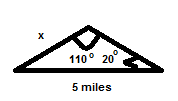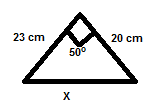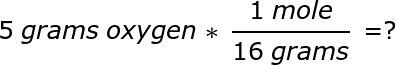Using Physics Formulas Chapter Exam

Exam Instructions:

Choose your answers to the questions and click 'Next' to see the next set of questions. You can skip questions if you would like and come back to them later with the yellow "Go To First Skipped Question" button. When you have completed the practice exam, a green submit button will appear. Click it to see your results. Good luck!

Page 1

Question 2 2. A student attaches a 5 N weight to a spring. The spring starts at 0.2m above the floor and ends at 0.15m above the floor. What is the spring constant?

Use this material to answer questions #3 through #4

Use the following scenario to answer questions:

A person weighing 70 kg drops from an airplane that is 1500 meters above the ground. After 15 seconds they have fallen 700 meters.

Question 3 3. How far would this person fall after the 15 seconds if they were falling in free fall?

Use this material to answer questions #3 through #4

Use the following scenario to answer questions:

A person weighing 70 kg drops from an airplane that is 1500 meters above the ground. After 15 seconds they have fallen 700 meters.

Question 4 4. What is the acceleration due to air resistance that this person is experiencing?

Use this material to answer question #5

You have piece of paper with two slits that are 2346 nm apart and each slit is 1546 nm. You want to create two dark spots that are 1.3 meters away from each other on a screen that is 5 meters away.

Page 2

Use this material to answer question #6

You are on an adventure and in order to open up a lock you need to shine a light into two spots that are exactly 2.3 meters apart. You need to stand 5 meters away from the spots. The flashlight that you have has a wavelength of 597 nm.

Question 6 6. What is the distance you'll need between the two slits to get the lights to shine properly?

Use this material to answer questions #7 through #8

Use the following scenario to answer the questions:

A 520 gram ball is held at chest height (1.2 meters) and then dropped. Recall that acceleration due to gravity is 9.8 m/s^2.

Question 7 7. What is the final velocity of the ball just before it lands on the ground?

Use this material to answer questions #7 through #8

Use the following scenario to answer the questions:

A 520 gram ball is held at chest height (1.2 meters) and then dropped. Recall that acceleration due to gravity is 9.8 m/s^2.

Page 3

Use this material to answer questions #11 through #12

Use the following spring to answer the questions. The spring can stretch out to 26 cm using 2 N of force. The highest amplitude it can stretch out to is 125 cm.

Question 11 11. What is the maximum possible potential energy of the spring?

Use this material to answer questions #11 through #12

Use the following spring to answer the questions. The spring can stretch out to 26 cm using 2 N of force. The highest amplitude it can stretch out to is 125 cm.

Question 13 13. What is the displacement of side x?Question 14 14. What is the displacement of the side x?Use this material to answer questions #15 through #16

You are swinging a ball (weighing 5 kg) around on a rope. The rope is 1.4 meters long. It takes 4 seconds for the ball to make one complete rotation.

Page 4

Use this material to answer questions #15 through #16

You are swinging a ball (weighing 5 kg) around on a rope. The rope is 1.4 meters long. It takes 4 seconds for the ball to make one complete rotation.

Page 5

Question 22 22. At a cookout, soda cans were submerged in a bucket of ice, which melted into water. The cans have a radius of 0.05m and are submerged 0.09m in the water. What is the buoyant force acting on the can?

Use this material to answer question #23

What is the height in meters of a 5.5 foot tall person? You are given the following information: 1 foot = 12 inches 1 inch = 2.54 centimeters 1 meter = 100 centimeters

Question 24 24. What are the final answer and units of this dimensional analysis equation?Page 6

Using Physics Formulas Chapter Exam Instructions

Choose your answers to the questions and click 'Next' to see the next set of questions. You can skip questions if you would like and come back to them later with the yellow "Go To First Skipped Question" button. When you have completed the practice exam, a green submit button will appear. Click it to see your results. Good luck!

Support